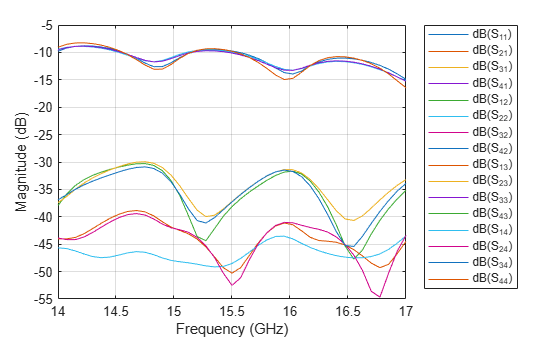# eggCrate

Create egg crate array of Vivaldi antenna elements

Since R2021a

## Description

The eggCrate object creates an array of Vivaldi antenna elements arranged in a rectangular egg-crate structure. Egg crate arrays are used in phased array applications in radar systems.## Creation

### Syntax

``array = eggCrate``
``array = eggCrate(Name=Value)``

### Description

example

``` `array = eggCrate` creates an array of Vivaldi antenna elements arranged in a rectangular egg-crate structure in the X-Y plane at an operating frequency of 825 MHz.```

example

``` `array = eggCrate(Name=Value)` creates an array of Vivaldi antenna elements, with additional Properties specified by one or more name-value arguments. `Name` is the property name and `Value` is the corresponding value. You can specify several name-value pair arguments in any order as `Name1=Value1`, `...`, `NameN=ValueN`. Properties you do not specify retain their default values.```

## Properties

expand all

Vivaldi antenna, specified as either a `vivaldi` or `vivaldiOffsetCavity` object.

Example: `vivaldi`

Number of rows and columns in the egg crate array, specified as a two-element vector.

Note

You can use NumElements to determines number of Vivaldi antenna elements in the egg-crate array.

Example: `[4 4]`

Data Types: `double`

Spacing between the Vivaldi antenna elements, specified as a two-element vector with each element in meters. The first element in the vector represents the spacing between the Vivaldi elements along x-axis. The second element represents the spacing between the Vivaldi elements along y-axis.

Example: `[0.1 0.2]`

Data Types: `double`

Magnitude of the voltage applied to the feed, specified as a as a positive scalar or a vector of positive elements in volts. If you specify a vector, it must be of the same size as `'NumElements'`.

Example: `2`

Data Types: `double`

Phase shift for each element in the array, specified as a real scalar or a vector of real elements in degrees. If you specify a vector, it must be of the same size as `'NumElements'`.

Example: `-12`

Data Types: `double`

Tilt angle of the array in degrees, specified as a scalar or vector. For more information, see Rotate Antennas and Arrays.

Example: `90`

Example: `Tilt=[90 90]`,`TiltAxis=[0 1 0;0 1 1]` tilts the array at 90 degrees about the two axes, defined by the vectors.

Data Types: `double`

Tilt axis of the array, specified as one of these values:

• Three-element vectors of Cartesian coordinates in meters. In this case, each vector starts at the origin and lies along the specified points on the x-, y-, and z-axes.

• Two points in space, specified as a 2-by-3 matrix corresponding to two three-element vectors of Cartesian coordinates. In this case, the array rotates around the line joining the two points.

• `"x"`, `"y"`, or `"z"` to describe a rotation about the x-, y-, or z-axis, respectively.

Example: `[0 1 0]`

Example: `[0 0 0;0 1 0]`

Example: `"Z"`

Data Types: `double` | `string`

Solver for antenna analysis, specified as either `"MoM"` (Method of Moments) or `"MoM-PO"` (Method of Moments-Physical Optics) hybrid or `"FMM"` (Fast Multipole Method).

Example: `"MoM-PO"`

Data Types: `string`

## Object Functions

 `show` Display antenna, array structures, shapes, or platform `solver` Access FMM solver for electromagnetic analysis `info` Display information about antenna or array `beamwidth` Beamwidth of antenna `charge` Charge distribution on antenna or array surface `current` Current distribution on antenna or array surface `efficiency` Radiation efficiency of antenna `EHfields` Electric and magnetic fields of antennas or embedded electric and magnetic fields of antenna element in arrays `impedance` Input impedance of antenna or scan impedance of array `mesh` Mesh properties of metal, dielectric antenna, or array structure `optimize` Optimize antenna or array using SADEA optimizer `pattern` Plot radiation pattern and phase of antenna or array or embedded pattern of antenna element in array `patternAzimuth` Azimuth plane radiation pattern of antenna or array `patternElevation` Elevation plane radiation pattern of antenna or array `rcs` Calculate and plot radar cross section (RCS) of platform, antenna, or array `returnLoss` Return loss of antenna or scan return loss of array `sparameters` Calculate S-parameters for antennas and antenna arrays `vswr` Voltage standing wave ratio (VSWR) of antenna or array element

## Examples

collapse all

Create a default egg-crate array.

`eca = eggCrate`
```eca = eggCrate with properties: Element: [1x1 vivaldi] Size: [2 2] Gap: [0 0] FeedVoltage: 1 FeedPhase: 0 Tilt: 0 TiltAxis: [1 0 0] SolverType: 'MoM' ```

View the array using the `show` function.

`show(eca)`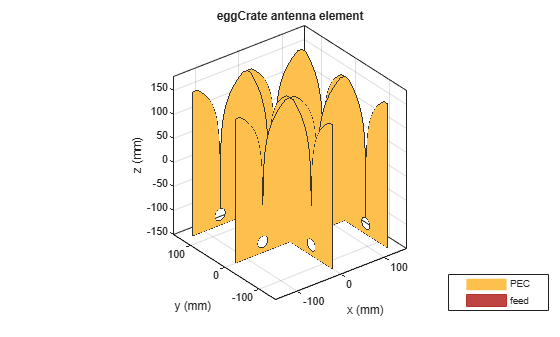Plot the radiation pattern of the egg-crate array at a frequency of 825 MHz.

`pattern(eca,825e6)`Create a 2-by-2 egg-crate array with a spacing of 20 mm between the Vivaldi elements.

`eca = eggCrate(Size=[2 2], Gap=[20e-3 20e-3])`
```eca = eggCrate with properties: Element: [1x1 vivaldi] Size: [2 2] Gap: [0.0200 0.0200] FeedVoltage: 1 FeedPhase: 0 Tilt: 0 TiltAxis: [1 0 0] SolverType: 'MoM' ```

View the array using the `show` function.

`show(eca)`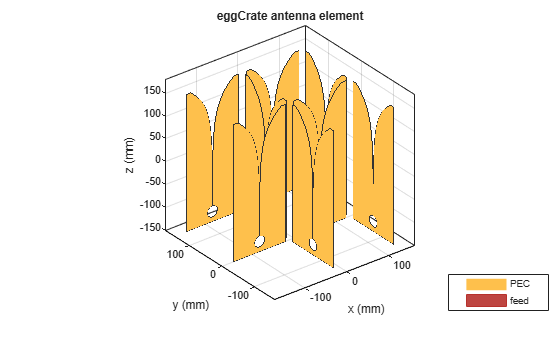Plot the radiation pattern of the array at a frequency of 0.9 GHz.

`pattern(eca,0.9e9)`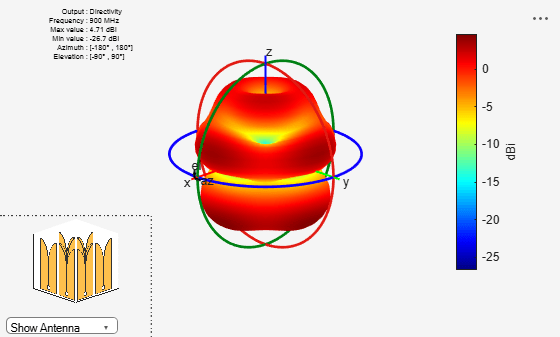Create a 1-by-4 egg-crate array with `vivaldiOffsetCavity` elements.

`eca = eggCrate(Element=vivaldiOffsetCavity, Size=[1 4])`
```eca = eggCrate with properties: Element: [1x1 vivaldiOffsetCavity] Size: [1 4] Gap: [0 0] FeedVoltage: 1 FeedPhase: 0 Tilt: 0 TiltAxis: [1 0 0] SolverType: 'MoM' ```

View array using the `show` function.

`show(eca)`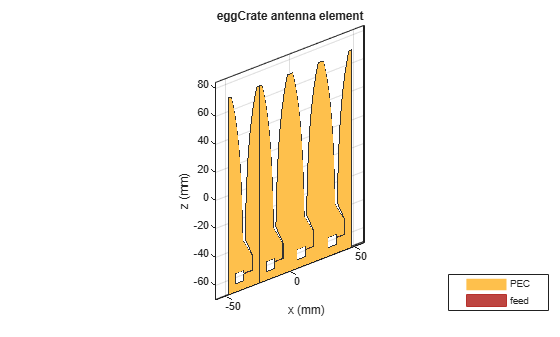Plot the S-parameters of the array over a frequency range of 14--17 GHz.

```s = sparameters(eca,linspace(14e9,17e9,41)); rfplot(s)```